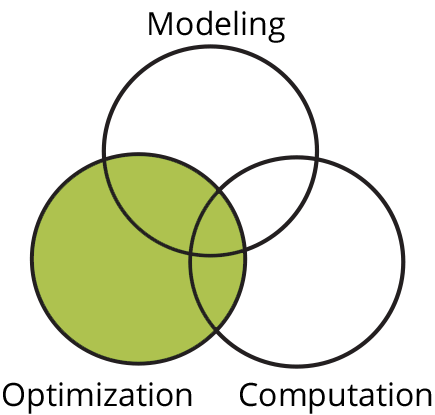# OptimizationWhether a mathematical model exists or one is inferred from data, it is often of critical interest to determine a set of problem parameters that optimize solution properties.  A goal of research in the area of optimization, and a second pillar of the MOCA center, is the design and analysis of algorithms that can find local or global optimizers to problems from models or data.

## Associated Projects

### First-order methods for structured large-scale problems

First-order methods (FOMs) or gradient-type methods find a solution of a problem by inquiring gradient and/or function value information. Compared to second-order or even higher-order methods, FOMs generally have much lower per-update complexity and much lower memory requirements.

### Rongjie's Project Page

The project introduction will be added shortly.

### Optimization problems with complementarity constraints

A complementarity constraint requires that one of a pair of variables should be zero. Optimization problems with complementarity constraints are widespread, arising for example in transportation problems, energy optimization, and sparse optimization.

### Rank minimization algorithms

We investigate the use of nonconvex approaches to rank minimization problems, an alternative to widely-used convex approaches such as nuclear norm minimization.